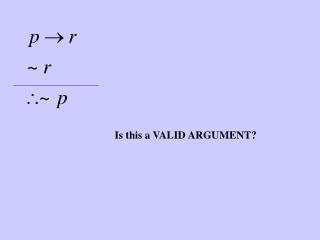DownloadDownload PresentationIs this a VALID ARGUMENT?

# Is this a VALID ARGUMENT?

Télécharger la présentation## Is this a VALID ARGUMENT?

- - - - - - - - - - - - - - - - - - - - - - - - - - - E N D - - - - - - - - - - - - - - - - - - - - - - - - - - -
##### Presentation Transcript

1. Is this a VALID ARGUMENT?

2. Place truth values under the conclusion

3. T T false If there is a row T T F it must be one of the first two rows The conclusion is false only if p is true

4. T T T T false Now place truth values under the second premise.

5. F F true If there is a row T T F then it must be the second row When the conclusion is false the second premise is true only if r is false T T T T false

6. false F In this case, we won’t find it because under these conditions the first premise is false T F F true T F T false We have eliminated all but one row in our search for the possibility T T F

7. T F false F true T F F T false VALID There are NO conditions under which the conclusion is false when the premises are true. If the premises are true then you must accept the conclusion.

8. Is this a VALID ARGUMENT?

9. Place truth values under the conclusion

10. F T T F F T false F T The conclusion is false only when r is trueand q is false

11. F T T F F T false F T Assign values to premise #1

12. F T T F F T false F T Assign values to premise #1

13. T F true T T T T F F T false F T When the conclusion is false, the first premise is true only when p is true

14. T F true T T false T T F F T F false VALID F T There are no conditions under which the conclusion is false and the premises are true.

15. Is this a VALID ARGUMENT?

16. Place truth values under the conclusion

17. The conclusion is false only if r is false

18. F F F F false F F The conclusion is false only if r is false

19. F F F F false F F Place truth values under premise #1

20. F F F F false F F When the conclusion is false premise #1 is true only when p is false

21. F F true F F F F false F F F F When the conclusion is false premise #1 is true only when p is false

22. F F true F F F F false F F F F If there is a row T T F then it is one of the yellow rows - complete them

23. F F true F T F true F F false F F T F F If there is a row T T F then it is one of the yellow rows - complete them

24. F F true F T F true F F false F F T invalid F F There are conditions under which the premises are true but the conclusion is false.

25. F F The conclusion is FALSE only if r is F F false

26. T Under these conditions, this premise is TRUE only if p is T T F true F F false

27. T T Under these conditions, this premise is TRUE only if qT true T T F true F F false

28. T T true F T T F true Under these conditions, this premise is TRUE only if s is F F F true F false

29. F T T true F T Under these conditions, this premise is FALSE T F true F F true F false

30. F T T It is not possible for all of the premises to be true when the conclusion is false. This is a valid argument. true F T false T F true F F true F false